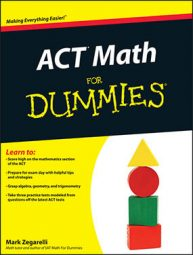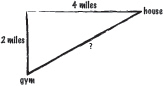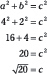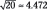##### ACT Math For DummiesSome math problems are difficult to visualize, so sketching out a chart or picture of the given information can help you arrive at the correct answer when taking the math portion of the ACT. Here are some tips to keep in mind:

• If you’re a visual person — an artist or a photographer, for example — start sketching sooner rather than later.

• Your sketch doesn’t have to be perfect. Just seeing how the question looks on paper may help you out.

• When you’ve got the beginnings of a sketch, step back from it and decide what kind of problem you’re trying to solve. For example, do you need arithmetic, algebra, or geometry to solve it?

Consider the following example question:

Jason likes to begin his workout with a run from his house to the gym. He runs 4 miles due west, then makes a left turn and runs 2 miles due south to arrive at the gym. Which of the following is the best approximation of the shortest distance from Jason’s house to the gym?

(A) 4.2 miles
(B) 4.5 miles
(C) 4.8 miles
(D) 5.2 miles
(E) 5.5 miles

At first reading, you may not see exactly what this question is asking. Making the following sketch helps put it into perspective:Now you can see that this problem is a geometry problem with a right triangle. You already know the lengths of the two legs, and you want to know the distance from the house to the gym, which is the hypotenuse. So use the Pythagorean theorem, as follows:Use your calculator to find thatSo the right answer is Choice (B).